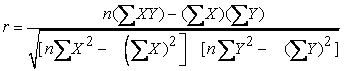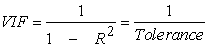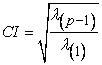﻿ Detecting Multicollinearity in Regression AnalysisPublications are Open
Access in this journal
Article Versions
Export Article
• Normal Style
• MLA Style
• APA Style
• Chicago Style
Research Article
Open Access Peer-reviewed

### Detecting Multicollinearity in Regression Analysis

Noora Shrestha
American Journal of Applied Mathematics and Statistics. 2020, 8(2), 39-42. DOI: 10.12691/ajams-8-2-1
Received May 05, 2020; Revised June 07, 2020; Accepted June 14, 2020

### Abstract

Multicollinearity occurs when the multiple linear regression analysis includes several variables that are significantly correlated not only with the dependent variable but also to each other. Multicollinearity makes some of the significant variables under study to be statistically insignificant. This paper discusses on the three primary techniques for detecting the multicollinearity using the questionnaire survey data on customer satisfaction. The first two techniques are the correlation coefficients and the variance inflation factor, while the third method is eigenvalue method. It is observed that the product attractiveness is more rational cause for the customer satisfaction than other predictors. Furthermore, advanced regression procedures such as principal components regression, weighted regression, and ridge regression method can be used to determine the presence of multicollinearity.

### 1. Introduction

In multiple regression analysis, the term multicollinearity indicates to the linear relationships among the independent variables. Collinearity indicates two variables that are close perfect linear combinations of one another. Multicollinearity occurs when the regression model includes several variables that are significantly correlated not only with the dependent variable but also to each other 1.

Multicollinearity is the event of great inter-correlations among the factors in a multiple regression model. Multicollinearity can prompt skewed or deluding results when an investigator endeavors to decide how well every factor can be utilized most viably to foresee or comprehend the response variable in a statistical model 2. All in all, multicollinearity can prompt more extensive confidence interval and less solid likelihood esteems for the predictors. That is, the findings from a model with multicollinearity may not be trustworthy 2, 3.

Sometimes adding more predictors to a regression analysis fail to give clear understanding of the model because of multicollinearity. The presence of multicollinearity increases the standard errors of each coefficient in the model, which in turn changes the result of the analysis. Multicollinearity makes some of the significant variables under study to be statistically insignificant 4. Multicollinearity increases variance of the regression coefficients making them unstable, which brings problem to interpret the coefficients 5. Several studies examined and discussed the problems of multicollinearity for regression model and also emphasized that the major problem related with the multicollinearity comprises uneven and biased standard errors and impractical explanations of the results 6, 7, 8. These studies also encourage researchers to consider the steps for detecting the multicollinearity in regression analysis. To determine the presence of multicollinearity, there are some more advanced regression procedures such as principal components regression, weighted regression, and ridge regression method 1, 8, 9.

The present study discusses on the three primary techniques for detecting the multicollinearity using the questionnaire survey data. The first two techniques, the correlation coefficients and variance inflation factor, are straightforward to implement while the third method is based on the eigenvalues and eigenvectors of the standardized design matrix 1, 5.

### 2. Materials and Methods

The structured questionnaire survey was conducted among 310 young smart phone users aged 18-27 years in October 2019. The research ethics committee of the educational institute in Kathmandu approved this study and the written consent of the respondent was taken prior. The respondents were asked to rate their view using Likert scale from 1 to 5 in some key areas. The major factors product quality, brand experience, product feature, product attractiveness, and product price that could potentially influence the level of satisfaction for smart phones were included in the survey questionnaire.

All the statistical procedures were performed using IBM SPSS version 23.0 for Mac. Scatterplot is constructed to display the relationship between the pair of variables. Pearson’s correlations were calculated for the relationship among the major factors. The regression analysis helps to provide an estimation of the relationship among the variables and the impact of independent variables on the outcome variables that exist within a population. Hence, the regression analysis was used for the variables of interest.

2.1. Techniques for Detecting Muticollinearity

The primary techniques for detecting the multicollnearity are i) correlation coefficient, ii) variance inflation factor, and iii) eigenvalue method.

2.1.1. Pairwise Scatterplot and Correlation Coefficients

The scatterplot is a graphical method that signifies the linear relationship between pairs of independent variables. It is important to look for any scatterplots that seem to indicate a linear relationship between pairs of independent variables.

The correlation coefficient is calculated using the formula:Where, r = correlation coefficient, n = number of observations, X = first variable in the context, and Y = second variable in the context. If the correlation coefficient value is higher with the pairwise variables, it indicates possibility of collinearity. In general, if the absolute value of Pearson correlation coefficient is close to 0.8, collinearity is likely to exist 1, 10.

2.1.2. Variance Inflation Factor (VIF)

Variance inflation factor is used to measure how much the variance of the estimated regression coefficient is inflated if the independent variables are correlated. VIF is calculated asWhere, the tolerance is simply the inverse of the VIF. The lower the tolerance, the more likely is the multicollinearity among the variables. The value of VIF =1 indicates that the independent variables are not correlated to each other. If the value of VIF is 1< VIF < 5, it specifies that the variables are moderately correlated to each other. The challenging value of VIF is between 5 to 10 as it specifies the highly correlated variables. If VIF ≥ 5 to 10, there will be multicollinearity among the predictors in the regression model and VIF > 10 indicate the regression coefficients are feebly estimated with the presence of multicollinearity 9.

2.1.3. Eigenvalue Method

Eigenvalue stands for the variance of the linear combination of the variables. Since the sum of eigenvalues (λ) must equal to the number of independent variables, the very small eigenvalue (close to 0.05) are indicative of multicollinearity and even small changes in the data lead to large changes in regression coefficient estimates. Sometimes it is difficult to interpret the eigenvalue close to 0.05 so conditional index can be the alternative. Moreover, condition index or condition number of the correlation matrix can be used to measure the overall multicollinearity of the variables. The condition index (CI) is a function of eigenvalues. It is given bywhere, (p – 1) is the number of predictors, , λ(p-1) and λ(1) are the maximum and minimum eigenvalues respectively. The value of CI is always greater than one, so a higher value of CI indicates the multicollinearity. Generally, CI <15 usually means weak multicollinearity, 15<CI<30 is evidence of moderate multicollinearity, and CI >30 is indication of strong multicollinearity 1, 11.

### 3. Results

The respondents were smartphone users between 18 to 27 years and living in acity. Out of total respondents, 45.8% were male and the rest 54.2% were female. The users were provided questionnaire to examine the predictors; product quality (PQ), brand experience (BE), product feature (PF), product attractiveness (PA), and product price (PP) that could potentially influence the level of customer satisfaction (CS) for smartphones.

3.1. Detecting Multicollinearity

Multicollinearity among the variables is examined using different methods.

3.1.1. Pairwise Scatterplot

A scatterplot is used to observe the relationship between the variables. It uses dots to represent values for two different variables. The location of each dot on the horizontal and vertical axis denotes values for an individual data point. Figure 1 shows a scatterplot of the data with the estimated simple linear regression line overlaid. It is useful to find outliers and observe the patterns between some dimensions. The figure shows positive correlation between the pairwise variables but does not give exact extent of correlation.

• Figure 1. Scatterplot of pairwise variables

3.1.2. Pearson’s Correlation Coefficients

Pearson’s correlation coefficient helps to check the collinearity of independent variables. Table 1 shows the correlation analysis between the customer satisfaction and other factors resulted with the moderate positive and significant correlation at p < 0.001.

The correlation coefficient of overall customer satisfaction with the variable brand experience has moderate correlation (r = 0.431, p < 0.01), followed by product attractiveness (r = 0.412, p < 0.01), product quality (r = 0.399, p < 0.01), product price (r = 0.368, p < 0.01), and product feature (r = 0.353, p < 0.01). Here the absolute value of Pearson correlation coefficient is less than 0.8, it shows collinearity is very less likely to exist.

3.1.3. Variance Inflation Factor (VIF)

Regression analysis is more applicable in the customer satisfaction survey because it helps to identify the factors that influence on customer satisfaction for smartphones. In Table 2, the multiple correlation coefficient between the independent variables and the outcome variable, customer satisfaction, is r = 0.570 which shows there is positive correlation between the variables. The R2 = 0.325 shows that 32.5% of the movement in the dependent variable can be explained by the independent variables and the rest 67.5% remains unexplained. The adjusted R2 = 0.316 gives the idea of how well the model generalizes. The difference between the R2 and adjusted R2 is 0.325-0.316= 0.009; it means if the model was derived from the population rather than a sample it would account for approximately 0.9% less variance the outcome. The F-value of ANOVA Table 3 measures the statistical significance of the model. Here, F-value is considered statistically significant at p < 0.001 and it can be observed that the outputs from the analysis are not due to chance alone.

The regression coefficient shows how much the dependent variable customer satisfaction is expected to increase when the predictor variable under consideration increases by one and all other independent variables are held at the same value. In regression analysis using stepwise method, it is observed that the variable product feature is not significant.

The value of VIF is 1< VIF < 5; it specifies that the variables are moderately correlated to each other. The small values of VIF corresponding to the variables show that there is no problem of collinearity.

3.1.4. Eigenvalue Method

Table 5 illustrates the eigenvalue, condition index and variance proportions. The dimension stands for the linear combination of the variables. Eigen value stands for the variance of the linear combination of the variables. The condition index is a function of eigenvalues. In Table 5 for variable brand experience, higher variance proportions i.e. 96% is associated with dimension 2 that has an eigenvalue of 0.039 and a condition index of 11.225. The variable product attractiveness has the higher variance of 75% and is associated with the dimension 3, with eigenvalue of 0.034 and a condition index of 11.89. Similarly, the higher variance of product quality and product-price are associated with the dimension 4 and 3 with eigenvalues 0.032 and 0.034 respectively. A condition index greater than 15 denotes a probable problem of multicollinearity. The higher condition index is 15.39 for dimension 5 but the variance proportions of variables are not associated with this value. This shows there is no evidence of collinearity among the variables.

In Table 4, the confidence interval shows the maximum and minimum values of the true value. The most likely true value of the coefficient for product attractiveness is 0.234. There is 95% level of confident that the true value lies between 0.123 and 0.345. The most likely true value of the coefficient for brand experience is 0.229. There is 95% level of confidence that the true value lies between 0.128 and 0.331 and so on. The confidence intervals of more than two regression coefficients are overlapped. It shows there is a chance that the true values of the coefficients of various variables will be of similar importance. The beta value (0.234) shows that product attractiveness is more rational cause than other three variables; brand experience, product quality, and product price. The variable product price is the less important cause for customer satisfaction. However, it is very difficult to identify the single most significant variable that influence the outcome because there is overlap of confidence intervals.

### 4. Conclusion

The relationship between customer satisfaction with the major factors product quality, brand experience, product feature, product attractiveness, and product price are significant with p<0.001. The multicollinearity among the variables is detected using the three techniques; correlation coefficients, variance inflation factor, and eigenvalue method. It is observed that there is no evidence of multicollinearity among the variables. The variable product attractiveness is the most significant variable that influences the outcome customer satisfaction.

Additionally, more extended researches including other significant variables can be conducted for identifying customer satisfaction. To determine the presence of multicollinearity, advanced regression procedures such as principal components regression, weighted regression, and ridge regression method can be used.

### References

  Young, D.S., Handbook of regression methods, CRC Press, Boca Raton, FL, 2017, 109-136. In article  Frank, E.H. Jr., Regression modeling strategies: with applications to linear models, logistic regression, and survival analysis, Springer, New York, 2001, 121-142. In article  Hosmer, D.W., Lemeshow, S. and Sturdivant, R.X., Applied Logistic Regression, John Wiley & Sons, New Jersey, 2013. In article View Article  Pedhajur, E.J., Multiple regression in behavioral research: explanation and prediction (3rd edition), Thomson Learning, Wadsworth, USA, 1997. In article  Keith, T.Z., Multiple regression and beyond: An introduction to multiple regression and structural equation modeling (2nd edition), Taylor and Francis, New York, 2015. In article View Article  Aiken, L.S. and West, S.G., Multiple regression: Testing and interpreting interactions, Sage, Newbury Park, 1991. In article  Gunst, R.F. and Webster, J.T., “Regression analysis and problems of multicollinearity,” Communications in Statistics, 4 (3). 277-292. 1975. In article View Article  Vatcheva, K.P., Lee, M., McCormick, J.B., and Rahbar, M.H., “Multicollinearity in regression analysis conducted in epidemiologic studies,” Epidemiology (Sunnyvale, Calif.), 6 (2). 227. 2016. In article  Belsley, D.A., Conditioning diagnostics: Collinearity and weak data in regression, John Wiley & Sons, Inc., New York, 1991. In article  Belinda, B. and Peat, J., Medical statistics: a guide to SPSS, data analysis, and critical appraisal (2nd edition), Wiley, UK, 2014. In article  Knoke, D., Bohrnstedt, W. G. and Mee, A. P., Statistics for social data analysis (4th edition), F.E. Peacock Publisher, Illinois, 2002. In articleThis work is licensed under a Creative Commons Attribution 4.0 International License. To view a copy of this license, visit http://creativecommons.org/licenses/by/4.0/